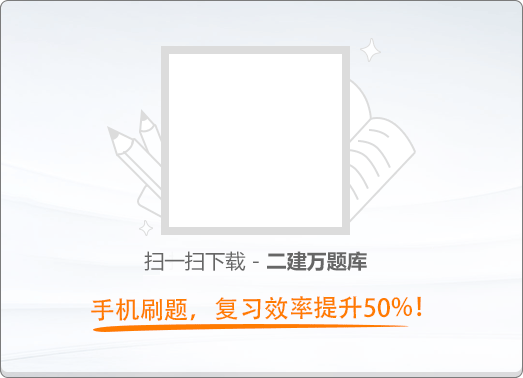180:002012年10月《建筑工程》真题

1
(单项选择题)

• A.

栏杆垂直杆件间的净距为100mm

• B.

临空高度25m部位，栏杆高度为1.05m

• C.

室外楼梯临边处栏杆离地面100mm高度内不留空

• D.

栏杆采用不易攀爬的构造

• A
• B
• C
• D

2
(单项选择题)

• A.

结构自重

• B.

雪荷载

• C.

风荷载

• D.

积灰荷载

• A
• B
• C
• D

3
(单项选择题)

• A.

高程控制网

• B.

城市控制网

• C.

轴线控制网

• D.

施工控制网

• A
• B
• C
• D

4
(单项选择题)

• A.

120mm厚墙

• B.

砌体门窗洞口两侧450mm处

• C.

独立柱

• D.

宽度为800mm的窗间墙

• A
• B
• C
• D

5
(单项选择题)

• A.

80%

• B.

85%

• C.

90%

• D.

95%

• A
• B
• C
• D

6
(单项选择题)

• A.

预埋件、钢筋吊杆进行了防锈处理

• B.

安装面板前完成吊顶内管道和设备的验收

• C.

检修口处未设置附加吊杆

• D.

距主龙骨端部300mm的部位设置了吊杆

• A
• B
• C
• D

7
(单项选择题)

• A.

严寒地区

• B.

寒冷地区

• C.

温和地区

• D.

炎热地区

• A
• B
• C
• D

8
(单项选择题)

• A.

悬挑脚手架工程

• B.

开挖深度为2.5m的基坑支护工程

• C.

采用常规起重设备，单件最大起重量为8kN的起重吊装工程

• D.

搭设高度10m的落地式钢管脚手架工程

• A
• B
• C
• D

9
(单项选择题)

• A.

所有用电设备用同一个专用开关箱

• B.

总配电箱无需加装漏电保护器

• C.

现场用电设备10台，编制了用电组织设计

• D.

施工现场的动力用电和照明用电形成一个用电回路

• A
• B
• C
• D

10
(单项选择题)

• A.

各道工序操作人员自检、互检和专职质量管理人员专检

• B.

各道工序操作人员自检、专职质量管理人员专检和监理人员专检

• C.

各道工序操作人员自检、互检和监理人员专检

• D.

各道工序操作人员互检、专职质量管理人员专检和监理人员专检

• A
• B
• C
• D

11
(单项选择题)

• A.

现场对螺栓孔采用气割扩孔

• B.

螺栓的紧固顺序从节点边缘向中间施拧

• C.

当天安装的螺栓均在当天终拧完毕

• D.

螺栓安装均一次拧紧到位

• A
• B
• C
• D

12
(单项选择题)

• A.

风压变形性能

• B.

水密性能

• C.

防火性能

• D.

气密性能

• A
• B
• C
• D

13
(单项选择题)

• A.

宽度为350mm的洞口上部未设置过梁

• B.

不同品种的水泥混合使用，拌制砂浆

• C.

混凝土小型空心砌块底面朝下砌于墙上

• D.

蒸压加气混凝土砌块龄期超过28天后砌筑

• A
• B
• C
• D

14
(单项选择题)

• A.

操作架子上长期堆放大量模板

• B.

高耸结构的模板作业设有避雷措施

• C.

遇到六级大风，停止模板安装作业

• D.

高压电线旁进行模板施工时，按规定采取隔离防护措施

• A
• B
• C
• D

15
(单项选择题)

• A.

照明变压器采用自耦变压器

• B.

易触及带电体的场所电源采用36V电压

• C.

人防工程施工照明电源采用24V电压

• D.

金属容器内的照明电源采用24V电压

• A
• B
• C
• D

16
(单项选择题)

• A.

预防为主、防消结合、综合治理

• B.

消防为主、防消结合、综合治理

• C.

安全第一、预防为主、综合治理

• D.

安全第一、防消结合、综合治理

• A
• B
• C
• D

17
(单项选择题)

• A.

房屋建筑的主体结构工程为30年

• B.

屋面防水工程为3年

• C.

电气管线、给排水管道为2年

• D.

装饰装修工程为1年

• A
• B
• C
• D

18
(单项选择题)

• A.

A级：不燃性

• B.

B级：阻燃性

• C.

C级：可燃性

• D.

D级：易燃性

• A
• B
• C
• D

19
(单项选择题)

• A.

住宅

• B.

学校教室

• C.

书店

• D.

医院

• A
• B
• C
• D

20
(单项选择题)

• A.

黄色

• B.

蓝色

• C.

蓝绿双色

• D.

黄绿双色

• A
• B
• C
• D